This post spoils a CTF challenge … Don’t read if you want to try it !

Sogeti CTF was a qualifier for the Sogeti Cyber E-Scape. I could not find a team to participate in this event, so I played alone and finally managed to find a team for the final :)

## [+] Presentation

Find the flag in this executable.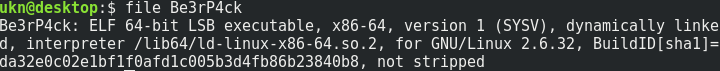## [+] Reversing 1

We are facing a simple program which asks us to input a flag.

After analyzing the program, it appears that our input is XORed with 0x87, and then NOTed 4 times.
Of course, not not not not something is something, so our input is simply XORed with 0x87.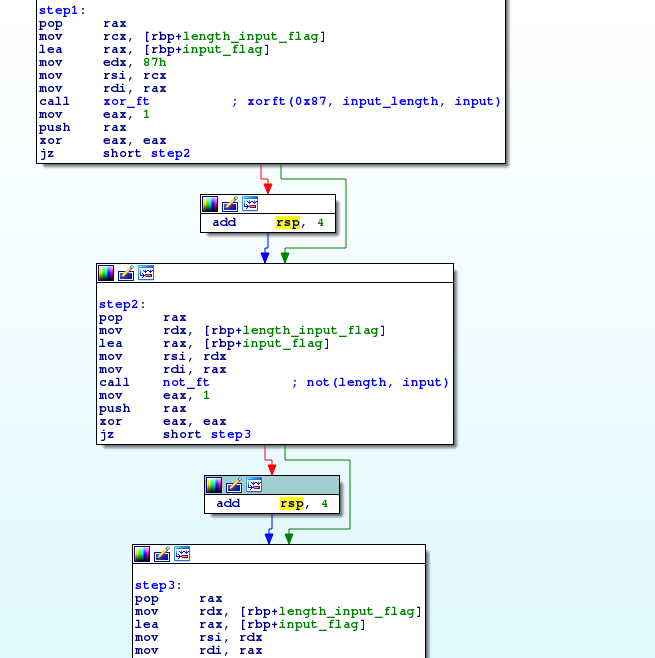The result is then compared with ‘\xc9\xb7\xf3\xd8\xd3\xef\xb4\xd8\xe1\xeb\xb3\xc0\xc0\xc0\xc0\xc0’.

Here is the flag … ?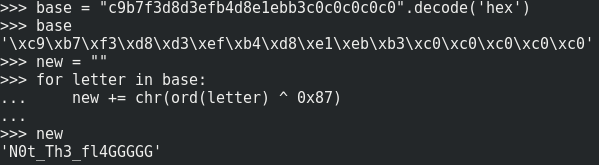Well, this is the wrong flag. The main function is not the real entry point of this program : there’s a function called main_0, which calls the previous main if the number of arguments provided to the program is different than 2.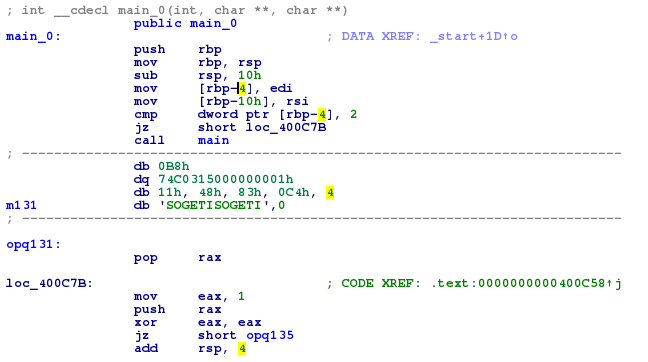So, let’s try to find this real program ;)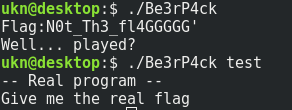The binary is obfuscated using overlapping instructions: there are some data bytes in the executable code, so disassemblers wrongly interpret the assembly instructions. Fortunately, IDA is good enough to detect the meaningful code.

After analysis, it appears that the current program contains a zlib compressed data block.

When you run the executable, this block is uncompressed and then xored with ‘ELF’.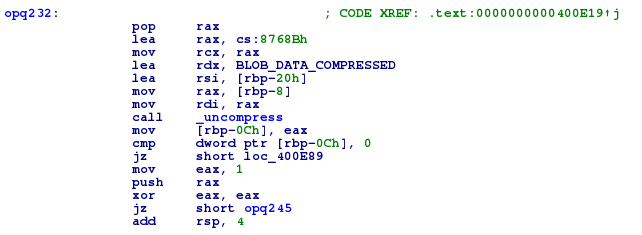The result of these operations is used to create a file in memory with the `memfd_create` function and then execute it with the arguments we gave to the first program.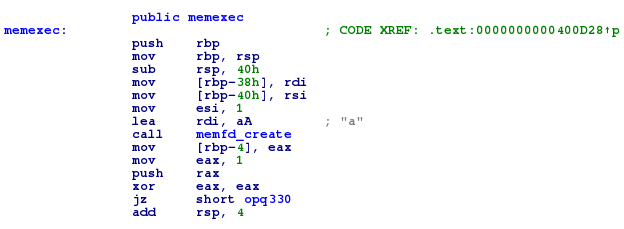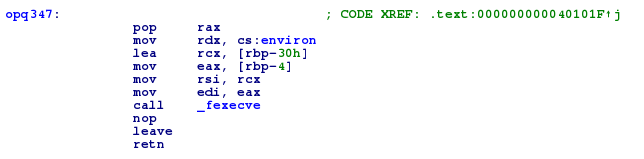Let’s dump the second executable with this python script :

``````#!/usr/bin/python

import zlib

with open('Be3rP4ck') as f:

dump = zlib.decompress(data[0x20c0:0x8974b])

dump2 = ""

for i in range(len(dump)):
dump2 += chr(ord(dump[i]) ^ ord("ELF"[i%3]))

with open('stage2.elf','w') as f:
f.write(dump2)
``````

## [+] Reversing 2

We now have our second stage, which is a Go binary ;)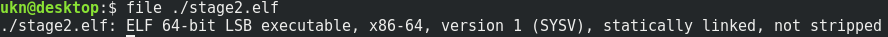It structure is simple, it shows us a welcome message, calculate a value based on our input and compare it to a reference string in the executable.
If both strings are equal, we win ;)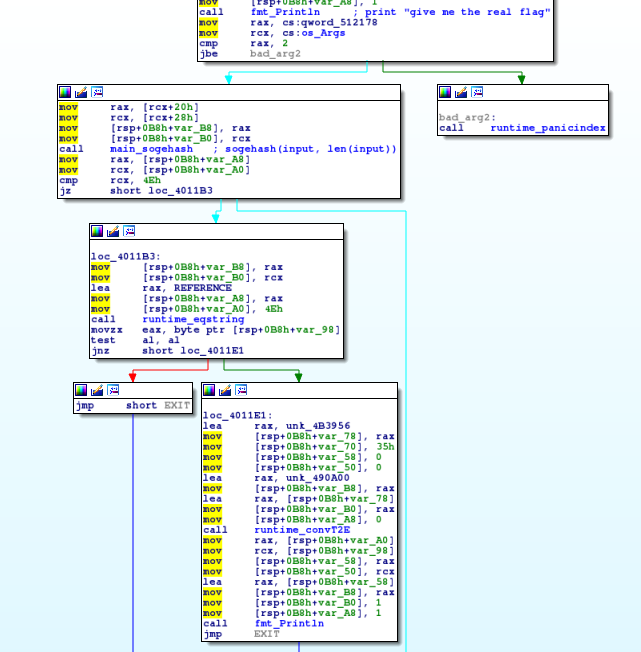The reference string is “1f4e509605c9f4bf22f4bf22a5c9fe23bbfee5dd22ffdde5fb22aafedcdd22f5f1d6f0a4a5a589” (hex encoded).
The custom “hash” function which computes the flag from our input is `sogehash`.

Here is the function code :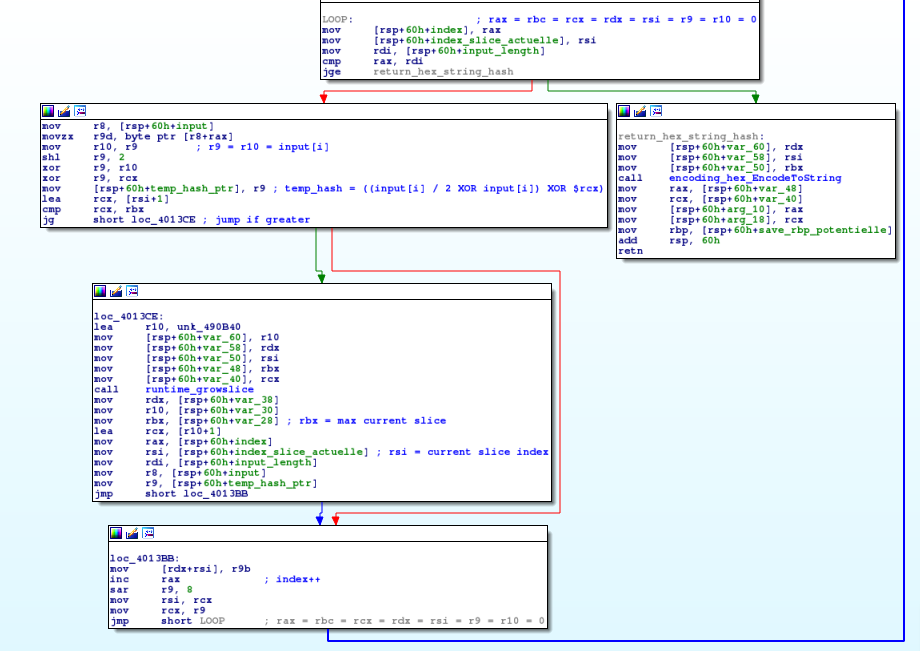Once reversed, the sogehash function is the following in Python :

``````def sogehash(REF):
prev = 0x00
out = ""
for char in REF:
new_char = ((ord(char) << 2) ^ ord(char))
out += "%x" % ((((ord(char) << 2) ^ ord(char)) ^ (prev >> 8)) & 0xff)
prev = new_char
return out
``````

We just have to reverse this function, and apply the reversed function to the reference string to get the flag !

``````def un_sogehash(REF, current = ""):
if len(current) == len(REF):
return current
elif len(current) == 0:
prev = 0x00
else:
prev = ((ord(current[-1]) << 2) ^ ord(current[-1]))
for char in string.printable:
new_char = ((((ord(char) << 2) ^ ord(char)) ^ (prev >> 8)) & 0xff)
if new_char == ord(REF[len(current)]):
new = un_sogehash(REF, current+char)
if new == False:
pass
else:
return new
return False
``````

Here’s the final script.

Flag : SCE{Th1s_1s_th3_r3al_fl4g_w3ll_d0ne!!!}

## [+] Bye

Feel free to tell me what you think about this post :)